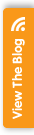# Algebra Help

### Information

##### Subject Areas
Maths,

An algebra based site that contains lessons, worksheets and more to help teachers and their students.

## Learning and Teaching Resources

Algebra Help provides a collection of materials and resources that can be used to help pupils learn about algebra. Algebra features in the Key Stage 3 National Curriculum maths programme of study and pupils should understand that it is represents generalised arithmetic. There are lots of lessons (with an index and descriptions), worksheets (with an index), calulators and other information.

There is a prime numbers list (that can show as many numbers as you wish to see) as well as study tips and also a squares chart that teachers and pupils can use in Maths classes along side the algebra topic. Study tips are for pupils who need help when learning and practising algebra and the equations.

### Content of the Site

There are suggested lessons on the basics of algebra, which outline key subject-knowledge and worked examples. This covers aspects of algebra such as equations, simplifying equations and factoring. These plans are laid out simply and contain examples through text and image. Each section is made clear so it is easy to understand.

### Worksheets

Online worksheets are available to download or be used on the actual site. Each problem has a step-by-step guide to arriving at the solution. These worksheets cover basic equations, basic proportion and word problems involving algebra. There are also worksheets on simplifying and other aspects of algebra. Pupils can fill in the problems on the site and check their answers. An answer sheet is supplied so that teachers can use it in lessons to either present examples or mark the pupils worksheets.

### Online Calculator

An online calculator for algebra can also be accessed. This allows equations to be entered and will be solved by the calculator. There is a guide to how these are entered and how symbols should be used when inputting equations. The calculator can be used in different areas of algebra.

### 0 Customer Star Ratings & Reviews## Newsletter Signup

regular updates about free resources

Sign up now to get regular updates about free resources for your subject